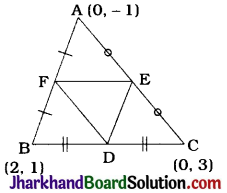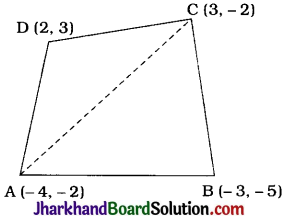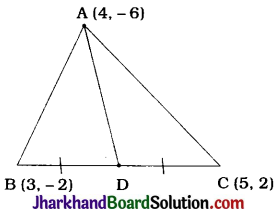# JAC Class 10 Maths Solutions Chapter 7 Coordinate Geometry Ex 7.3

Jharkhand Board JAC Class 10 Maths Solutions Chapter 7 Coordinate Geometry Ex 7.3 Textbook Exercise Questions and Answers.

## JAC Board Class 10 Maths Solutions Chapter 7 Coordinate Geometry Exercise 7.3

Question 1.
Find the area of the triangle whose vertices are :
1. (2, 3), (-1, 0), (2, -4)
2. (5, 1), (3, 5), (5, 2)
1. Let A (2, 3), B(-1, 0) and C(2, – 4) be the vertices of ΔABC. Then, using the formula, the area of ΔABC is given by $$\frac{1}{2}$$[2 (0 + 4) + (-1) (- 4 – 3) + 2(3 – 0)]
= $$\frac{1}{2}$$(8 + 7 + 6)
= $$\frac{21}{2}$$ sq units
Thus, the area of the triangle with given vertices is $$\frac{21}{2}$$ sq units.

2. The vertices of the triangle are given to be (-5, -1), (3, – 5) and (5, 2).
Then, using the formula, the area of the triangle is given by
= $$\frac{1}{2}$$[-5(-5 – 2) + 3(2 + 1) + 5 (-1 + 5)]
= $$\frac{1}{2}$$(35 + 9 + 20)
= $$\frac{1}{2}$$(64)
= 32 sq units
Thus, the area of the triangle with given vertices is 32 sq units.Question 2.
In each of the following find the value of ‘k’, for which the points are collinear:
1. (7, -2), (5, 1), (3, k)
2. (8, 1), (k, – 4), (2, – 5)
1. If the points (7, -2), (5, 1) and (3, k) are collinear, then the area of imaginary triangle formed by those points as vertices must be zero.
∴ $$\frac{1}{2}$$[7 (1 – k) + 5 (k + 2) + 3(- 2 – 1)] = 0
∴ $$\frac{1}{2}$$[7 – 7k + 5k + 10 – 9] = 0
∴ – 2k + 8 = 0
∴ 2k = 8
∴ k = 4

2. If the points (8, 1), (k, – 4) and (2, – 5) are collinear, then the area of imaginary triangle formed by those points as vertices must be zero.
∴ $$\frac{1}{2}$$[8 (- 4 + 5) + k(-5 – 1) + 2(1 + 4)] = 0
∴ 8 – 6k + 10 = 0
∴ 18 = 6k
∴ k = 3

Question 3.
Find the area of the triangle formed by joining the midpoints of the sides of the triangle whose vertices are (0, – 1), (2, 1) and (0, 3). Find the ratio of this area to the area of the given triangle.Let A (0, – 1), B (2, 1) and C(0, 3) be the vertices of ΔABC and let D, E and F be the midpoints of the sides BC, CA and AB respectively.
Then, coordinates of D = ($$\frac{2+0}{2}, \frac{1+3}{2}$$) = (1, 2)
Coordinates of E = ($$\frac{0+0}{2}, \frac{3-1}{2}$$) = (0, 1)
Coordinates of F = ($$\frac{0+2}{2}, \frac{-1+1}{2}$$) = (1, 0)
Area of ΔDEF
= $$\frac{1}{2}$$[1(1 – 0) + 0(0 – 2) + 1 (2 – 1)]
= $$\frac{1}{2}$$(1 + 0 + 1)
= 1 sq unit
Area of ΔABC
= $$\frac{1}{2}$$[0(1 – 3) + 2(3 + 1) + 0 (- 1 – 1)]
= $$\frac{1}{2}$$(0 + 8 + 0)
= 4 sq units
Then, area of ΔDEF : area of ΔABC = 1 : 4.
Thus, the area of triangle formed by joining the midpoints of the sides of the triangle with given vertices is 1 sq unit and the required ratio of areas is 1 : 4.

Question 4.
Find the area of the quadrilateral whose vertices, taken in order, are (-4, – 2), (- 3, – 5), (3, – 2) and (2, 3).Let A(- 4, – 2), B(- 3, – 5), C(3, – 2) and D (2, 3) be the vertices of quadrilateral ABCD.
Drawing diagonal AC, we get two triangles ABC and ADC.
The vertices of ΔABC are A (-4, – 2), B(-3, 5) and C(3, -2).
Area of ΔABC
= $$\frac{1}{2}$$[-4(-5 + 2) + (-3) (-2 + 2) + 3(-2 + 5)]
= $$\frac{1}{2}$$[12 + 0 + 9]
= $$\frac{21}{2}$$ sq units
The vertices of ΔADC are A(-4, -2), D(2, 3) and C(3, -2).
= $$\frac{1}{2}$$[- 4(3 + 2) + 2(- 2 + 2) + 3(- 2 – 3)]
= $$\frac{1}{2}$$(-20 + 0 – 15)
= $$\frac{1}{2}$$(- 35)
But area of a triangle cannot be negative.
Hence, we take the numerical value.
∴ Area of ΔADC = $$\frac{35}{2}$$sq units.
= area of ΔABC + area of ΔADC
= ($$\frac{21}{2}=\frac{35}{2}$$) sq units
= 28 sq units
Thus, the area of the given quadrilateral is 28 sq units.Question 5.
You have studied in Class IX, that a median of a triangle divides it into two triangles of equal areas. Verify this result for ΔABC whose vertices are A(4, 6), B (3, – 2) and C (5, 2).A (4, – 6) , B (3, – 2) and C (5, 2) are the vertices of ΔABC.
Let AD be a median of ΔABC.
Then, D is the midpoint of BC.
∴ Coordinates of D are ($$\frac{3+5}{2}, \frac{-2+2}{2}$$), ie., (4, 0)
Area of ΔABC
= $$\frac{1}{2}$$[4(- 2 – 2) + 3(2 + 6) + 5(- 6 + 2)]
= $$\frac{1}{2}$$[-16 + 24 – 20]
= $$\frac{1}{2}$$ (-12)
= 6 sq units (∵ Area cannot be negative.) ………(1)
Vertices of ΔADB are A (4, – 6), D (4, 0) and B (3, -2)
= $$\frac{1}{2}$$[4(0 + 2) + 4(- 2 + 6) + 3(- 6 – 0)]
= $$\frac{1}{2}$$[18 + 16 – 18]
= 3 sq units ……(2)

Vertices of ΔADC are A (4, – 6), D (4, 0) and C (5, 2).
= $$\frac{1}{2}$$[4 (0 – 2) + 4 (2 + 6) + 5 (- 6 – 0)]
= $$\frac{1}{2}$$[- 8 +32 – 30]
= $$\frac{1}{2}$$[-6]
Area of ΔADB = Area of ΔADC = $$\frac{1}{2}$$ Area of ΔABC